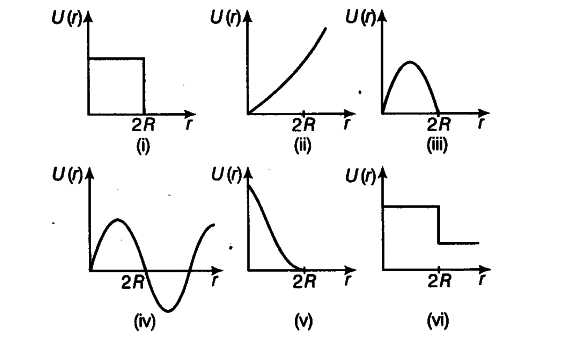# Which of the following potential energy curves in figure

Which of the following potential energy curves in figure cannot possibly describe the elastic collision of two billiard balls? Here r is the distance between centres of the balls.When two billiard balls collide then distance between their centres is 2R. Due to impact of collision there is small temporary deformation of balls. In this process, KE of ball is gradually reduced to zero and converted into elastic potential energy of balls. When KE is zero, the balls regain their original configuration due to elasticity. The phenomenon can be successfully explained only by potential energy curve number (v), because here as r < 2R, the potential energy function U{r) is increasing gradually oh decreasing value of r.